• Design and Analysis of Algorithms
• Home

Design and Analysis Shortest Paths

Dijkstra’s Algorithm

Dijkstra’s algorithm solves the single-source shortest-paths problem on a directed weighted graph G = (V, E), where all the edges are non-negative (i.e., w(u, v) ≥ 0 for each edge (u, v) Є E).

In the following algorithm, we will use one function Extract-Min(), which extracts the node with the smallest key.

Algorithm: Dijkstra’s-Algorithm (G, w, s)
for each vertex v Є G.V
v.d := ∞
v.∏ := NIL
s.d := 0
S := Ф
Q := G.V
while Q ≠ Ф
u := Extract-Min (Q)
S := S U {u}
for each vertex v Є G.adj[u]
if v.d > u.d + w(u, v)
v.d := u.d + w(u, v)
v.∏ := u

Analysis

The complexity of this algorithm is fully dependent on the implementation of Extract-Min function. If extract min function is implemented using linear search, the complexity of this algorithm is O(V2 + E).

In this algorithm, if we use min-heap on which Extract-Min() function works to return the node from Q with the smallest key, the complexity of this algorithm can be reduced further.

Example

Let us consider vertex 1 and 9 as the start and destination vertex respectively. Initially, all the vertices except the start vertex are marked by ∞ and the start vertex is marked by 0.

Vertex Initial Step1 V1 Step2 V3 Step3 V2 Step4 V4 Step5 V5 Step6 V7 Step7 V8 Step8 V6
1 0 0 0 0 0 0 0 0 0
2 5 4 4 4 4 4 4 4
3 2 2 2 2 2 2 2 2
4 7 7 7 7 7 7
5 11 9 9 9 9 9
6 17 17 16 16
7 11 11 11 11 11 11 11
8 16 13 13 13
9 20

Hence, the minimum distance of vertex 9 from vertex 1 is 20. And the path is

1→ 3→ 7→ 8→ 6→ 9

This path is determined based on predecessor information.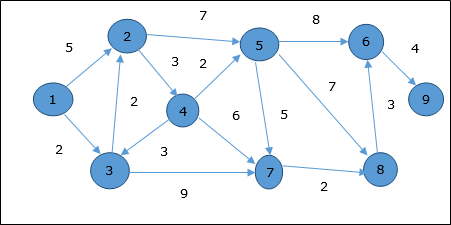Bellman Ford Algorithm

This algorithm solves the single source shortest path problem of a directed graph G = (V, E) in which the edge weights may be negative. Moreover, this algorithm can be applied to find the shortest path, if there does not exist any negative weighted cycle.

Algorithm: Bellman-Ford-Algorithm (G, w, s)
for each vertex v Є G.V
v.d := ∞
v.∏ := NIL
s.d := 0
for i = 1 to |G.V| - 1
for each edge (u, v) Є G.E
if v.d > u.d + w(u, v)
v.d := u.d +w(u, v)
v.∏ := u
for each edge (u, v) Є G.E
if v.d > u.d + w(u, v)
return FALSE
return TRUE

Analysis

The first for loop is used for initialization, which runs in O(V) times. The next for loop runs |V - 1| passes over the edges, which takes O(E) times.

Hence, Bellman-Ford algorithm runs in O(V, E) time.

Example

The following example shows how Bellman-Ford algorithm works step by step. This graph has a negative edge but does not have any negative cycle, hence the problem can be solved using this technique.

At the time of initialization, all the vertices except the source are marked by ∞ and the source is marked by 0.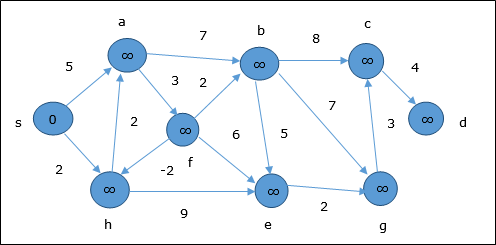In the first step, all the vertices which are reachable from the source are updated by minimum cost. Hence, vertices a and h are updated.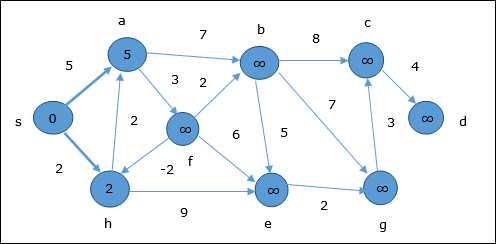In the next step, vertices a, b, f and e are updated.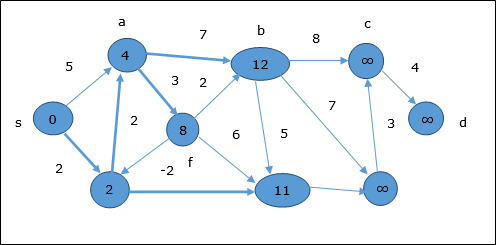Following the same logic, in this step vertices b, f, c and g are updated.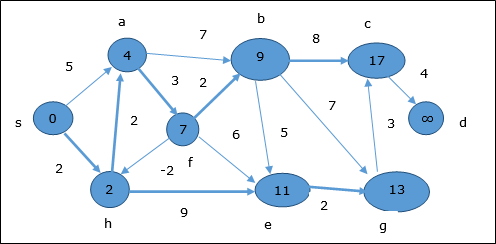Here, vertices c and d are updated.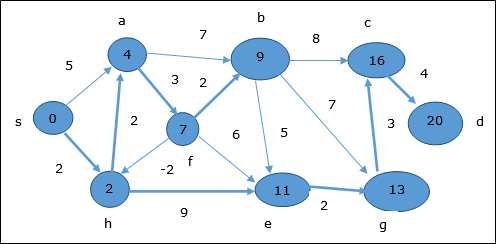Hence, the minimum distance between vertex s and vertex d is 20.

Based on the predecessor information, the path is s→ h→ e→ g→ c→ d

Useful Video Courses

Video

Compiler Design Online Training

102 Lectures 10 hours

Video

Sequential Circuit Design

30 Lectures 3 hours

Video

Design Patterns Online Training

31 Lectures 4 hours

Video

InDesign Online Training

43 Lectures 1.5 hours

Video

Canva: Become a Graphic Designer

7 Lectures 1 hours

Video

The Ultimate Canva Graphic Design Course

54 Lectures 4 hours